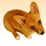# Calculate Field - VB - Conditional If,elseif statement... not working?!

7989
7
05-04-2012 07:37 AMNew Contributor III
Hi,

Wihtin model builder I have used the field calculator, to try and populate a new empty field (called Water_Classification) based on the contents of another field. However, my VB statement is not working and I am unable to figure out why? If anybody can help, it would be much appreciated 🙂

I would like to put numeric values in a field called [Water_Classification] depending on what the string values are from another field called [CLASSIFICATION_1]

This is what I currently have:

If [CLASSIFICATION_1] = "High" then
x =5
ElseIf [CLASSIFICATION_1] = "Medium" then
x =3
ElseIf [CLASSIFICATION_1] = "Low" then
x =1
ElseIf [CLASSIFICATION_1] = "<null>" then
x =0
Else
x = 9999
EndIf

At the end (in the box at the bottom), I have: Water_Classification = x

If anybody has any advice or tips, pelase let me know,
Thanks!
Tags (3)
1 Solution

Accepted SolutionsbyMVP Honored Contributor
"EndIf" needs a space ---------> "end if"

Also, IsNull is a function, and requires ()'s around the field name.
7 RepliesbyMVP Notable Contributor
I think you need to add at the top of your code a simple dim statement like:

Dim x

Also not sure this line is correct:

ElseIf [CLASSIFICATION_1] = "<null>"

you may want to try something like (I did not test these):

ElseIf [CLASSIFICATION_1] = ""

or

ElseIf IsNull([CLASSIFICATION_1]) = True

DuncanNew Contributor III
Hhmm I have added Dim x at the top and changed that one line to be ElseIf IsNull([CLASSIFICATION_1]) = True then.... However its still not working. But I am getting a new error, which says "Error Executing Funtion. Expected 'Then' Failed to execute".
Thanks for the help! any other ideas?byMVP Notable Contributor
Can you post up the script, exactly how you entered it?New Contributor III
Dim x
If [CLASSIFICATION_1] = "High" then
x =5
ElseIf [CLASSIFICATION_1] = "Medium" then
x =3
ElseIf [CLASSIFICATION_1] = "Low" then
x =1
ElseIf IsNull [CLASSIFICATION_1] = True then
x=0
Else
x = 9999
EndIf

Water_Classification= x

[ATTACH=CONFIG]14100[/ATTACH]

[ATTACH=CONFIG]14101[/ATTACH]byMVP Honored Contributor
"EndIf" needs a space ---------> "end if"

Also, IsNull is a function, and requires ()'s around the field name.byMVP Notable Contributor
This is going to be painful...

EndIf

should be (note space)

End If

DuncanNew Contributor III
You guys are BRILLIANT !!
Both the space was required and the ()'s after IsNull.

Thank you so much!

Hailey